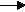gcsescience.com                                          28                                          gcsescience.com

Acids and Alkalis

What is an Ionic Equation?

If a reaction involves substances that form ions in water, we can write
the equation showing only the ions. This is called an ionic equation.

What is
the Ionic Equation for Neutralisation?

In the reaction between hydrochloric acid and sodium hydroxide
(see the previous page)

H
Cl(aq)       +          NaOH(aq)NaCl(aq)      +       H2O(l)

We can write it in terms of ions, since both
hydrochloric acid and sodium hydroxide form ions in water.

H+(aq) + Cl-(aq)Na+(aq)OH-(aq)Na+(aq)Cl-(aq)  +  H2O(l)

Chloride ions
(Cl-(aq)), and sodium ions (Na+(aq)) appear on
both sides of the equation. They are not changed by the reaction
(see spectator ions) and so they may be left out of the equation.

This leaves the ionic equation

hydrogen ion     +     hydroxide ionwater
H+(aq)            +         OH-(aq)H2O(l)

(compare this reaction with the ionisation of water).

This is the reaction that always occurs when
an acid + alkalisalt + water.
It is called the
ionic equation for
neutralisation.

The hydrogen ion of the acid + the hydroxide ion of the alkali
combine to form a water molecule,
leaving the metal from the alkali and the non-metal from
the
acid to form a
salt solution.

How much
acid is needed to neutralise an alkali?
(see the
next page).

gcsescience.com    The Periodic Table    Index    Neutralisation Quiz    gcsescience.com

Copyright © 2015 gcsescience.com. All Rights Reserved.